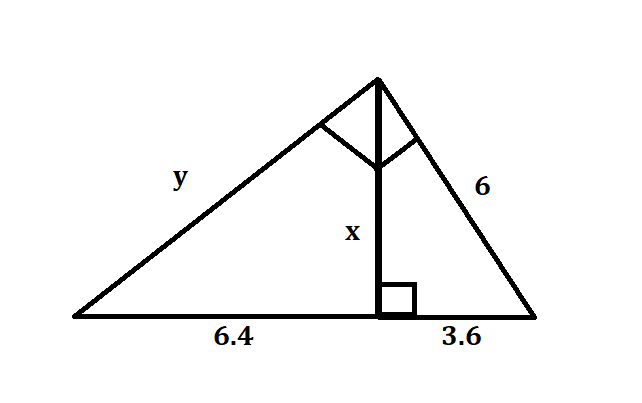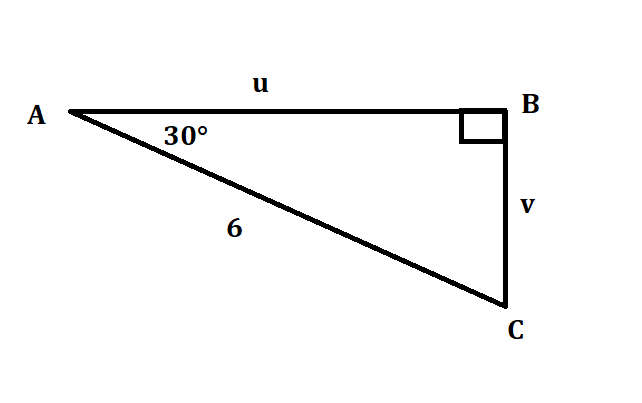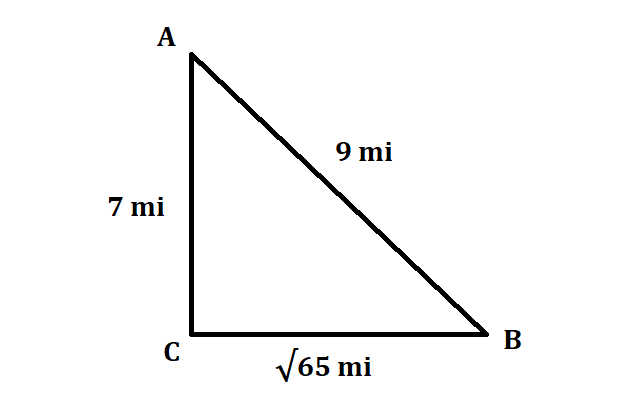# Find the values of the variables in each right triangle. 01510201141.pngQuestion
Right triangles and trigonometryFind the values of the variables in each right triangle.2021-03-10
Apply Pythagoras theorem, to find the lengths of x and y.
Pythagoras Theorem states that in a right-angled triangle sum of squares of perpendicular adjacent sides of a triangle is equal to the square of the third side of the triangle.
In the smaller right-angled triangle:
$$\displaystyle{x}^{{2}}+{\left({3.6}\right)}^{{2}}={6}^{{2}}$$
$$\displaystyle{x}^{{2}}={36}-{12.96}$$
$$\displaystyle{x}^{{2}}={23.04}$$
$$\displaystyle{x}={4.8}$$
In the larger right-angled triangle:
$$\displaystyle{y}^{{2}}+{6}^{{2}}={\left({6.4}+{3.6}\right)}^{{2}}$$
$$\displaystyle{y}^{{2}}+{6}^{{2}}={10}^{{2}}$$
$$\displaystyle{y}^{{2}}={100}-{36}$$
$$\displaystyle{y}^{{2}}={64}$$
$$\displaystyle{y}={8}$$
Thus, the values of x and y are 4.8 and 8 respectively.

### Relevant QuestionsFind the values of the variables in each right triangle.Label the words of this 30,60,90 right triangle $$\displaystyle{S}{L},{2}{S}{L},{\quad\text{and}\quad}{S}{L}\sqrt{{2}}$$Label the words of this 30,60,90 right triangle $$\displaystyle{S}{L},{2}{S}{L},{\quad\text{and}\quad}{S}{L}\sqrt{{2}}$$Label the words of this 30,60,90 right triangle $$\displaystyle{S}{L},{2}{S}{L},{\quad\text{and}\quad}{S}{L}\sqrt{{2}}$$Is this triangle a right triangleIs this triangle a right triangleIs this triangle a right triangleUse function notation to describe the way the second variable (DV) depends upon the first variable (IV). Determine the domain and range for each, determine if there is a positive, negative, or no relationship, and explain your answers.
A)IV: an acute angle V in a right triangle: DV: the area B of the triangle if the hypotenuse is a fixed length G.
B)IV: one leg P of a right triangle: DV: the hypotenuse G of the right triangle if the other leg is 2
C)IV: the hypotenuse G of a right triangle: DV: the other leg P of the right triangle is one leg is 5.